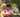### Quick Sort

January 18, 2020

Quick Sort

Quicksort algorithm picks a pivot element, rearranges the array elements in such a way that all elements smaller than the picked pivot element move to left side of pivot, and all greater elements move to right side. Finally, the algorithm recursively sorts the subarrays on left and right of pivot element.

Steps:
1. Remove the last item & use it as the pivot item.
2. Iterate and compare the array items with pivot : if equal or smaller, add the items to left. Otherwise, to the right array.
3. Return new array concat() with recursive calls

``````const quickSort = (A) => {
if(A.length <= 1) return A
var left = []
var right = []
var newA = []
var pivot = A.pop() // remove the last element
for(var i=0; i<A.length; i++){
if(A[i] <= pivot) left.push(A[i])
else right.push(A[i])
}
return newA.concat(quickSort(left), pivot, quickSort(right))
}

function qsort(A){
console.log("source: ", A)
console.log("sorted: ", quickSort(A))
}

qsort([3, 0, 2, 5, -1, 4, 1])``````

QuickSort Algorithm in JavaScript

Default sort() in JavaScript uses insertion sort by V8 Engine of Chrome and Merge sort by Mozilla Firefox and Safari. But not suitable if you need to sort large number of elements. So, the solution is to use Quick sort for large dataset.

### What is Quick sort

Quick sort follows Divide and Conquer algorithm. It is dividing elements in to smaller parts based on some condition and performing the sort operations on those divided smaller parts. Hence, it works well for large datasets. So, here are the steps how Quick sort works in simple words.

• First select an element which is to be called as pivot element.
• Next, compare all array elements with the selected pivot element and arrange them in such a way that, elements less than the pivot element are to it's left and greater than pivot is to it's right.
• Finally, perform the same operations on left and right side elements to the pivot element.

### Steps to perform Quick sort

Here are the steps to perform Quick sort that is being shown with an example [5,3,7,6,2,9].

STEP 1: Determine pivot as middle element. So, 7 is the pivot element.

STEP 2: Start left and right pointers as first and last elements of the array respectively. So, left pointer is pointing to 5 at index 0 and right pointer is pointing to 9 at index 5.

STEP 3: Compare element at the left pointer with the pivot element. Since, 5 < 6 shift left pointer to the right to index 1.

STEP 4: Now, still 3 <6 so shift left pointer to one more index to the right. So now 7 > 6 stop incrementing the left pointer and now left pointer is at index 2.

STEP 5: Now, compare value at the right pointer with the pivot element. Since 9 > 6 move the right pointer to the left. Now as 2 < 6 stop moving the right pointer.

STEP 6: Swap both values present at left and right pointers with each other.

STEP 7: Move both pointers one more step.

STEP 8: Since 6 = 6, move pointers to one more step and stop as left pointer crosses the right pointer and return the index of the left pointer.

``````const swap = (items, leftIndex, rightIndex) => {
var temp = items[leftIndex];
items[leftIndex] = items[rightIndex];
items[rightIndex] = temp;
}

const partition = (items, left, right) => {
var pivot   = items[Math.floor((right + left) / 2)] //middle element
i       = left, //left pointer
j       = right; //right pointer

while (i <= j) {  // until left index cross right index
while (items[i] < pivot) { // move left pointer to right until the value >= pivot
i++;
}
while (items[j] > pivot) { // move right pointer to left until the value <= pivot
j--;
}
if (i <= j) { // if left index <= right index, makes the swap
swap(items, i, j); // sawpping left (larger) and right (smaller)
i++;
j--;
}
}

return i; // return left index
}

const quickSort = (items, left, right) => {
var index;
if (items.length > 1) { // not empty
index = partition(items, left, right); //left index returned from partition
if (left < index - 1) { //more elements on the left side of the pivot
quickSort(items, left, index - 1);
}
if (index < right) { //more elements on the right side of the pivot
quickSort(items, index, right);
}
}
//console.log("quickSort returns: ", items)
return items;
}

function qsort(items){
console.log("\nsource ***: ", items)
console.log("sorted ***: ", quickSort(items, 0, items.length-1))
}

qsort([8, 4, 9, 2, 1, 5, 7])
qsort([9, 4, 6, 2, -1, 5, 7, 2])``````Posted by Tai Lu ( 呂台生 ) who's building useful things.You should follow him on Twitter